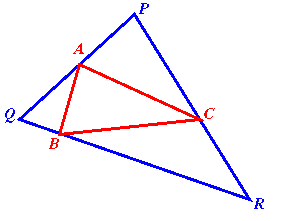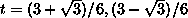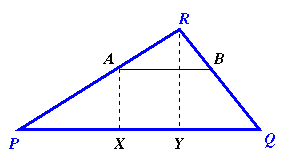#### You may also like### Areas and Ratios

Do you have enough information to work out the area of the shaded quadrilateral?### Napoleon's Hat

Three equilateral triangles ABC, AYX and XZB are drawn with the point X a moveable point on AB. The points P, Q and R are the centres of the three triangles. What can you say about triangle PQR?### Plane to See

P is the midpoint of an edge of a cube and Q divides another edge in the ratio 1 to 4. Find the ratio of the volumes of the two pieces of the cube cut by a plane through PQ and a vertex.

# Halving the Triangle

##### Age 16 to 18Challenge Level

Only one successful solution to this thought provoking problem was submitted, another triumph for the stars at Madras College, St Andrews. This was from Luke Halliwell, Alex Godwin and Neil Donaldson, all in S6.

The solution given below is essentially the same as the solution form Madras College.If A, B and C are midpoints of the sides of triangle PQR than the triangle ABC has one quarter the area of triangle PQR.

The next problem is to find an inscribed triangle which has half the area of triangle PQR.

Triangle PQR has area K and sides p , q , r (according to the usual convention) so that
K = 1/2 pq sinR.

Take points on the sides of the triangle dividing the sides in the same ratio such that:
A splits PQ into lengths t r , (1 - t ) r
B splits QR into lengths t p , (1 - t ) p
C splits RP into lengths t q , (1 - t ) q

We want to find a value for t such that area ABC = K/2.

Area RBC = 1/2 [(1 - t ) p ][ tq ] sinR = t (1 - t )K

Similarly, area QAB = t (1 - t )K
and area PCA = t (1 - t )K.

Hence, area PQR = K - 3 t (1- t )K = (3 t ² -3 t +1)K.

To find the required value of t we solve the equation 3 t ² -3 t +1 = 1/2

i.e. 6 t ² - 6 t + 1 = 0

givingNote that the sum of the roots is 1 so there is essentially one answer, the second root merely giving the ratio taking points P, Q and R anti-clockwise rather than clockwise.

Consider the quadratic polynomial:
f(t) = 3 - 3 t + 1,
for t between 0 and 1, which gives the ratio of the areas of the inscribed triangle ABC and triangle PQR. For t =0 and t =1 this ratio is 1 (as expected because for these values of t the triangles co-incide). The minimum value occurs when t = 1/2, in which case the inscribed circle has one quarter the area of the large triangle. By continuity then we see that, choosing equal ratios, it is possible to find a value of t such that the ratio of the areas has any value between 1/4 and 1.

Another way to look at this is to consider the quadratic equation:
3 t ² - 3 t + 1 = s
(where s is the ratio of the areas ABC to PQR, for 0 < s < 1).

The equation 3 t ²-3 t +(1- s )=0 has discriminant 12 s -3 so it has real solutions for s >= 1/4. We have to look for another method for ratios less than 1/4.

#### Fractions less than one quarter:For an arbitrary t (0 < t < 1) we take points A and B such that AB is parallel to PQ and t is the linear scale factor of the enlargement between the similar triangles RAB and RPQ. We shall use that PA = (1 - t )PR. Taking X and Y to be the feet of the perpendiculars from A and from R to PQ, then AX is the height of triangle ABC (with AB as base) and AX = (1- t )RY.

The ratio of the areas of triangles ABC and PQR is then:
Area ABC = ½AB· AX = ½ t PQ·(1- t )RY = t (1- t ) Area PQR.

For some fraction s (0 < s < 1) we solve
t ² - t + s = 0 .

This equation has discriminant 1 - 4 s so there are real solutions for s < 1/4 and this construction gives a method for 0 < s < 1/4.

For s = 1/4 the equation above has equal roots giving A, B and C as midpoints of the sides of PQR as before.